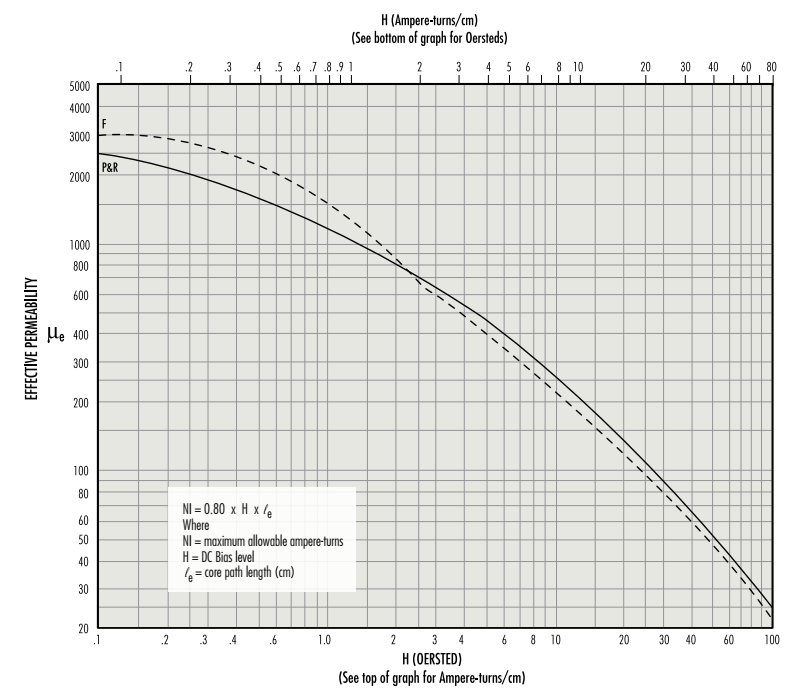# Inductor Design with Magnetics Ferrite Cores

The following design guide may also be downloaded as a PDF. For other inquiries regarding inductor design with Magnetics ferrite cores, Contact our Applications Engineers or submit a Custom Inductor Design request.

Ferrite E cores and pot cores offer the advantages of decreased cost and low core losses at high frequencies. For switching regulators, power materials are recommended because of their temperature and DC bias characteristics. By adding air gaps to these ferrite shapes, the cores can be used efficiently while avoiding saturation.

These core selection procedures simplify the design of inductors for switching regulator applications. One can determine the smallest core size, assuming a winding factor of 50% and wire current carrying capacity of 500 circular mils per ampere.

Only two parameters of the design applications must be known:
(a) Inductance required with DC bias
(b) DC current

1. Compute the product of LI² where:
L = inductance required with DC bias (millihenries)
I = maximum DC output current + 1/2 AC Ripple

2. Locate the LI² value on the Ferrite Core Selector chart below.
Follow this coordinate in the intersection with the first core size curve. Read the maximum nominal inductance, AL, on the Y-axis. This represents the smallest core size and maximum AL at which saturation will be avoided.

3. Any core size line that intersects the LI² coordinate represents a workable core for the inductor if the core’s AL value is less than the maximum value obtained on the chart.

4. Required inductance L, core size, and core nominal inductance (AL) are known.
Calculate the number of turns usingwhere L is in millihenries.

5. Example: If IMAX = 8 Amps; L, inductance required = 100 μHenries
LI² = (0.100 mH) X (8² Amps) = 6.4 millijoules

6. There are many ferrite cores available that will support the energy required.
Any core size that the LI² coordinate intersects can be used at the AL value shown on the chart.

7. Some choices based upon an LI² value of 6.4 millijoules are:
Pot core 43622 AL = 400      Double Slab 43622 AL = 250
PQ core 43220 AL = 300       E core 44317 AL = 250

8. For the following AL values the number of turns required is:
AL = 400, N = 16       AL = 300, N = 19       AL = 250, N = 20
Make sure the wire size chosen will support the current and fit into the core set.The above curves represent the locus of points up to which effective permeability remains constant. They show the maximum allowable DC bias, in ampere-turns, without a reduction in inductance. Beyond this level, inductance drops rapidly.

Example: How many ampere-turns can be supported by an 0R42213A315 pot core without a reduction in inductance value?
le = 3.12 cm μe = 125

Maximum allowable H = 25 Oersted (from the graph above)
NI (maximum) = 0.80 x H x le = 62.4 ampere-turns
or (Using top scale, maximum allowable H = 20 A•T/cm)
NI (maximum) = A•T/cm x le
= 20 x 3.12
= 62.4 A•T